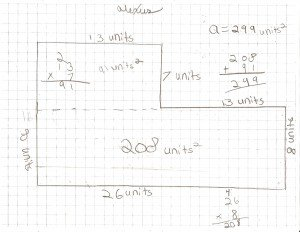Select Page
Read the Full Post Below

# Finding Area

Feb 5, 2011 | 54 comments

We’ve been learning about how to find the area of rectangles in math. In order to find the area, you must multiply the length and the width.In the rectangle above, we see that the length is 8 inches and the width is 6 inches. In order to find the area, you must multiply these together: 8 x 6 = 48. Therefore, our area is 48 square inches. We say square inches because you could draw squares inside of your rectangle that are an inch on each side. If you counted up all the squares you made, you’d have 48 of them!

Sometimes, you may find an irregular shape (such as a room in a house). In order to find the area, you have to split it up into separate rectangles. Find the area of each rectangle (length x width). Then, add those together. That will give you the total area of the shape!In the example above, you can see that the polygon is not a rectangle. So, we need to draw a line to make rectangles (dotted green line). To find the area of the larger rectangle, we multiply the length x width: 8 feet x 8 feet = 64 square feet. Then, we find the area of the smaller rectangle: 5 feet x 4 feet = 20 square feet. To find the total area, we add those together: 64 square feet + 20 square feet = 84 square feet!

Here’s another example:To help us better understand how to find the area of different shapes, Jenna and Ben from Avery Realty, created a video. They’ll share with you how you could find the area of rectangular and irregular shaped rooms in a house.
Did Ben and Jenna’s video help you understand area?

Can you find the area of anything else besides a room?

## Subscribe By Email

Get a weekly email of all new posts.

Please prove that you are not a robot.